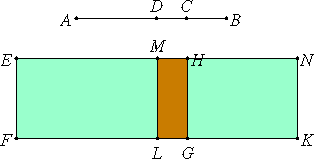# Proposition 44

A second bimedial straight line is divided at one point only.
X.38

Let AB be a second bimedial straight line divided at C, so that AC and CB are medial straight lines commensurable in square only and containing a medial rectangle. It is then manifest that C is not at the point of bisection, because the segments are not commensurable in length.

I say that AB is not so divided at another point.X.41.Lemma

If possible, let it also be divided at D, so that AC is not the same with DB, but AC is supposed greater. It is then clear that the sum of the squares on AD and DB is also, as we proved above, less than the sum of the squares on AC and CB. Suppose that AD and DB are medial straight lines commensurable in square only and containing a medial rectangle.

II.4

Set out a rational straight line EF, apply to EF the rectangular parallelogram EK equal to the square on AB, and subtract EG, equal to the sum of the squares on AC and CB. Then the remainder HK equals twice the rectangle AC by CB.

X.41.Lemma

Again, subtract EL, equal to the sum of the squares on AD and DB, which were proved less than the sum of the squares on AC and CB. Then the remainder MK also equals twice the rectangle AD by DB.

Now, since the squares on AC and CB are medial, therefore EG is medial.

X.22

And it is applied to the rational straight line EF, therefore EH is rational and incommensurable in length with EF.

For the same reason HN is also rational and incommensurable in length with EF.

And, since AC and CB are medial straight lines commensurable in square only, therefore AC is incommensurable in length with CB.

X.11

But AC is to CB as the square on AC is to the rectangle AC by CB, therefore the square on AC is incommensurable with the rectangle AC by CB.

X.15

But the sum of the squares on AC and CB is commensurable with the square on AC, for AC and CB are commensurable in square.

X.6

And twice the rectangle AC by CB is commensurable with the rectangle AC by CB.

X.13

Therefore the sum of the squares on AC and CB is also incommensurable with twice the rectangle AC by CB.

But EG equals the sum of the squares on AC and CB, and HK equals twice the rectangle AC by CB, therefore EG is incommensurable with HK, so that EH is also incommensurable in length with HN.

And they are rational, therefore EH and HN are rational straight lines commensurable in square only.

X.36

But, if two rational straight lines commensurable in square only are added together, then the whole is the irrational which is called binomial.

Therefore EN is a binomial straight line divided at H.

In the same way EM and MN is also proved to be rational straight lines commensurable in square only, and EN is a binomial straight line divided at different points, H and M.

And EH is not the same with MN, for the sum of the squares on AC and CB is greater than the sum of the squares on AD and DB.

But the sum of the squares on AD and DB is greater than twice the rectangle AD by DB, therefore the sum of the squares on AC and CB, that is, EG, is much greater than twice the rectangle AD by DB, that is, MK, so that EH is also greater than MN.

Therefore EH is not the same with MN.

Therefore, a second bimedial straight line is divided at one point only.

Q.E.D.

## Guide

This proposition is not used in the rest of the Elements.